Courses

# Notes | EduRev

## JEE : Notes | EduRev

The document Notes | EduRev is a part of the JEE Course JEE Main Mock Test Series 2020 & Previous Year Papers.
All you need of JEE at this link: JEE

Question 1: The gas liberated on heating a mixture of two salts with NaOH gives a reddish brown precipitate with an alkaline solution of K2HgI4. The aqueous solution of the mixture on treatment with BaCl2 gives a white precipitate which is sparingly soluble in conc. HCl. On heating the mixture with K2Cr2O7 and conc. H2SO4 red vapours of A are produced. The aqueous solution of the mixture gives a deep blue colouration B with potassium ferricyanide solution. Identify the radicals in the given mixture and write the balanced equations for the formation of A and B.
Solution: Let us summarise the given facts of the question.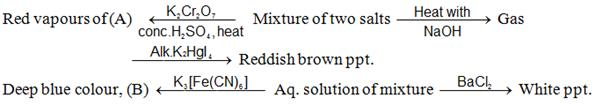The given reactions lead to following conclusions.
(i) Heating of mixture with NaOH to give NHgas (indicated by reddish brown ppt. with alkaline solution of K2HgI4) indicates the presence of ion in the mixture
(ii) Heating of mixture with K2Cr2O7 and conc. H2SO4 to give red vapours (of chromyl chloride) indicates the presence of Cl ion in the mixture.
(iii) Reaction of aqueous solution of the mixture with barium chloride solution to give white ppt. (of BaSO4) sparingly soluble in conc. HCl indicates the presence of  ions in the mixture.
(iv) Reaction of aqueous solution of the mixture with potassium ferricyanide solution to give deep blue colour indicates the presence of Fe2+ ions in the mixture.
Hence the mixture contains the following four ions. NH4+, Fe2,  SO42-and Cl.
Equations for the formation of A and B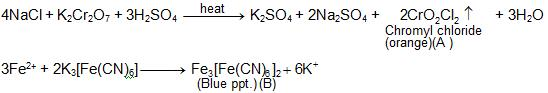Question 2: When an orange coloured crystalline compound (A) was heated with common salt and concentrated sulphuric acid an orange - yellow coloured gas (B) was evolved. The gas (B) when passed through caustic soda solution gave a yellow solution (C) which in turn gave the following reactions.
1. Addition of silver nitrate solution to (C) gave first a white precipitate which then turns red. Quantitatively, 0.155 g of the gas (B) required 2.0 m moles of AgNO3 to produce the first trace of red colour.
2. Acidification of the solution (C) with dil. H2SO4 gave an orange solution which contained chromium in +6 oxidation state. The solution liberated iodine from aqueous potassium iodide, leaving a green solution containing chromium in +3 oxidation state. Quantitatively 0.155 g of the gas (B) liberated 1.5 mmole of iodine.
Deduce the formula of A, B and C and explain the reactions.
Solution: Let us summaries the given reactions.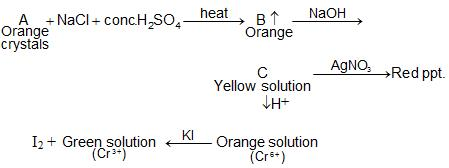The above set of reactions indicates that compound A is K2Cr2O7, B is chromyl chloride gas and C is sodium chromate which explains all the given reactions as below.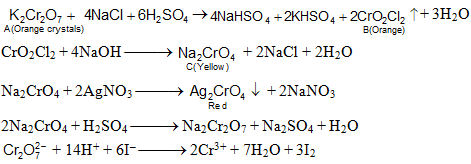The quantitative data is explained in the following manner. From the above reactions we observe that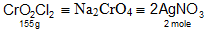155 g of CrO2Cl require 2 mole of AgNO3
This also coincides with the given data.

Question 3: A white amorphous powder (A) when heated, gives a colourless gas (B), which turns lime water milky (which dissolves on passing excess of gas (B) and the  residue (C) which is yellow while hot but white when cold. The residue (C) dissolves in dilute HCl and the resulting solution gives a white precipitate on addition of potassium ferrocyanide solution. (A) dissolves in dil. HCl with the evolution of a gas which is identical in all respects to gas (B). The solution of (A) in dil. HCl gives a white ppt. (D) on addition of NH4Cl excess of NH4OH and on passing H2S gas. Another portion of this solution gives initially a white ppt. (E) on addition of NaOH  solution which dissolves in excess of NaOH. The solution on passing again H2S gives back the white ppt. of (E), the white ppt. on heating with dil H2SO4 give a gas used in II and IV group analysis. What are (A) to (E)? Give balanced chemical equations of the reactions.
Solution: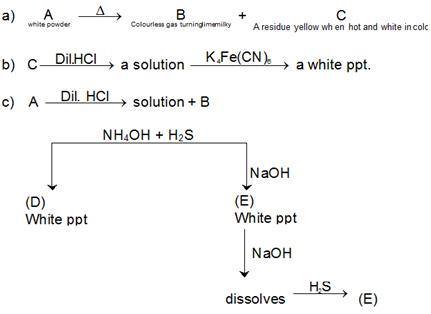From observations for section (a), one may conclude that the colourless gas is CO2 because it turns lime water milky, due to formation of insoluble CaCO3.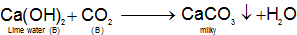CaCO3  is soluble in excess of CO2 due to formation of soluble calcium bicarbonate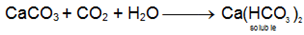The compound (C) is zinc oxide (ZnO) because it is yellow when hot and white when cold, hence the initial compound (A) is zinc carbonate (ZnCO3).
From section (b), it is inferred that (C) is a salt of Zn(II) which dissolves in dil. HCl and white ppt. obtained after addition of K4Fe(CN)6 is due to zinc ferrocyanid.
ZnCObecause on treatment with dil. HCl it gives gas (B), i.e., CO2, while Zn (II) goes in solution, i.e., ZnCl2. On passing H2S gas in presence of NH4OH, it gives a white ppt. of ZnS(D). ZnS on heating with dil. H2SO4 evolves H2S, which is used for the precipitation of sulphides of group II in acidic medium and of IV group in alkaline medium. ZnCl2, reacts with NaOH to give a ppt. of  Zn(OH)2 which dissolves in NaOH, as Zn(OH)2 is amphoteric in nature. The solution Na2ZnO2 again gives ZnS on passing H2S gas into it. Different chemical equations concerned are given below: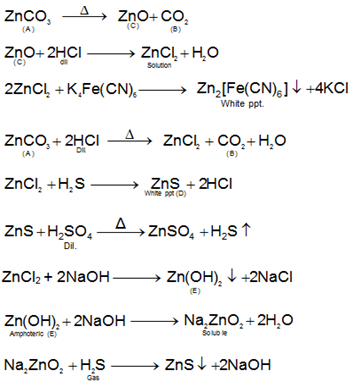Offer running on EduRev: Apply code STAYHOME200 to get INR 200 off on our premium plan EduRev Infinity!

## JEE Main Mock Test Series 2020 & Previous Year Papers

3 videos|174 docs|151 tests

,

,

,

,

,

,

,

,

,

,

,

,

,

,

,

,

,

,

,

,

,

;